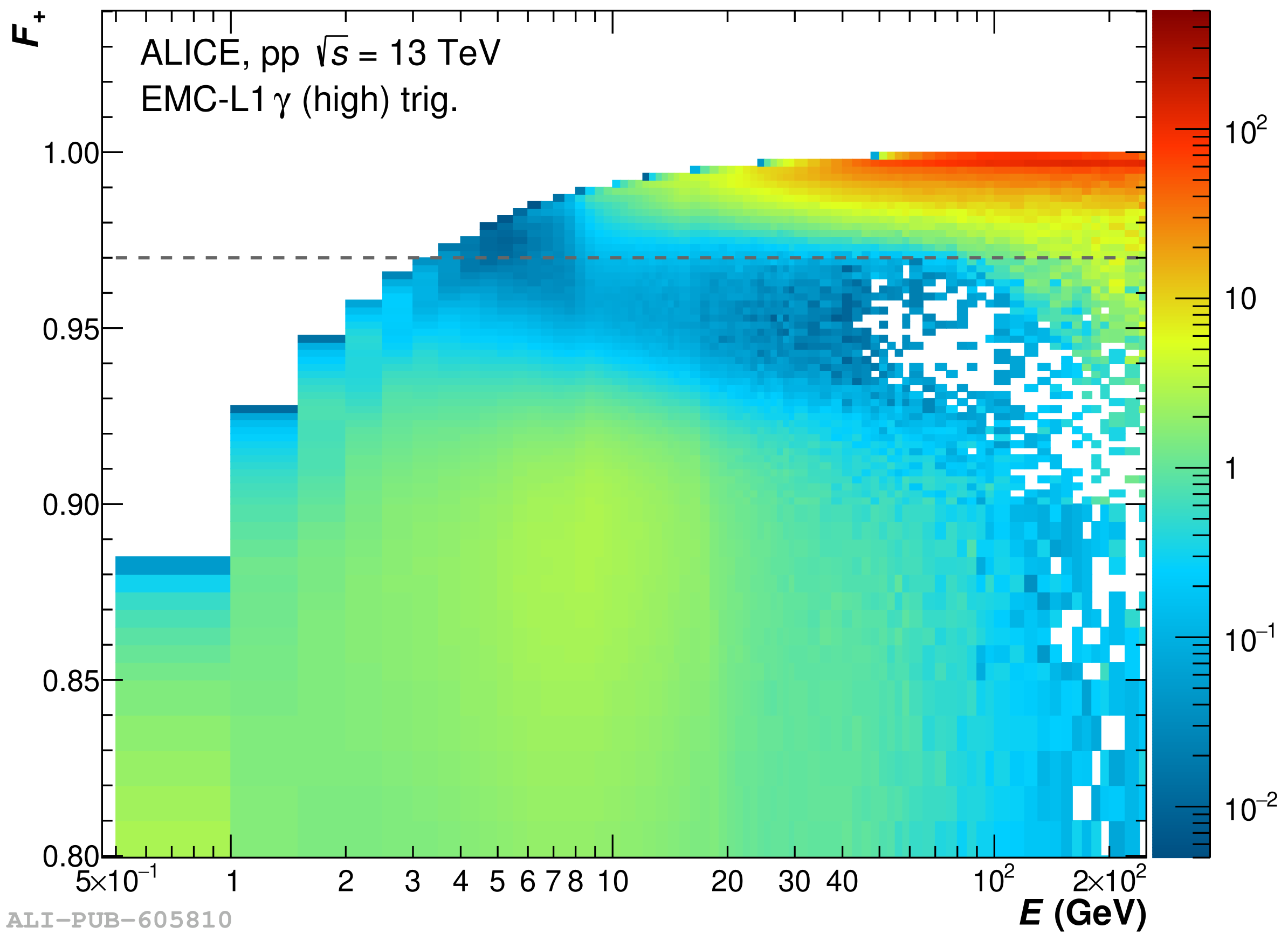# Figure 16

 Left: exoticity'' ($F_{\rm +}$) as function of the cluster energy found with the V2 clusterizer in \pp\ collisions at \sthirteen\ using the \gls{EMCal} high threshold \gls{L1} $\gamma$ trigger The region above the line is populated by exotic clusters The distributions are normalized to have an integral of unity for each energy bin. Right: comparison of $F_{+}$ probability distributions for measured data (black), projection of the left panel, and simulated (blue) collisions for two different cluster-energy intervals. Each distribution is normalized by the integral of the distribution for $F_{+}<0.85$ .